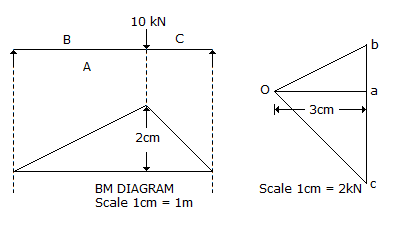# Civil Engineering - UPSC Civil Service Exam Questions

31.

The defect which develops due to uncontrolled and non-uniform loss of moisture from wood is known as which one of the following ?

 A. Kont B. Shake C. Warping D. Cross grain

Explanation:

No answer description available for this question. Let us discuss.

32.

Consider the following statements in respect of the critical depth of flow in a prismatical rectangular channel:
1. For known specific emergy, discharge is minimum.
2. For known discharge, the specific energy is minimum.
Which of the statements given above is/are correct ?

 A. 1 only B. 2 only C. Both 1 and 2 D. neither 1 nor 2

Explanation:

No answer description available for this question. Let us discuss.

33.

On the basis of the data given in the following figures, the bending moment under the load would work out toA. 2 kN.m B. 4 kN.m C. 6 kN.m D. 12 kN.m

Explanation:

No answer description available for this question. Let us discuss.

34.

What is the innermost portion of approach zone which is the most critical portion from obstruction viewpoint, called ?

 A. Outer horizontal surface B. Conical surface C. Inner horizontal surface D. Clear zone

Explanation:

No answer description available for this question. Let us discuss.

35.

A circular plate 100 mm diameter is welded to another plate by means of 6 mm fillet weld. If the permissible shearing stress in the weld equals 10 kg/mm2, then the greatest twisting moment that can be resisted by the weld will be

 A. 424 π kg.m B. 300 π kg.m C. 212 π kg.m D. 60 π kg.m